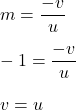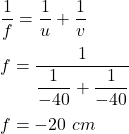## a spherical mirror produces a magnification of -1 on a screen placed at a distance of 40cm from the mirror i) write the type of mirror ii) W

Question

a spherical mirror produces a magnification of -1 on a screen placed at a distance of 40cm from the mirror i) write the type of mirror ii) What is the focal length of the mirror

in progress 0
6 months 2021-07-29T02:31:14+00:00 1 Answers 7 views 0

f =-20 cm

Explanation:

Given that,

The magnification of a spherical mirror, m = -1

The image distance, v = 40 cm (for negative magnification)

The magnification of a concave mirror is negative. The mirror showing -1 magnification is a concave mirror.

Let f be the focal length of the mirror. We know that,Object distance, u = -40 cm

Using mirror’s formula i.e.So, the focal length of the mirror is 20 cm.